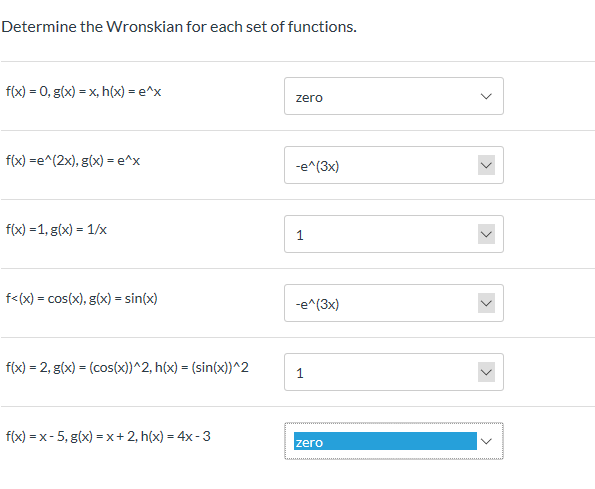# Determine the Wronskian for each set of functions.fx) 0, g(x)x, h(x) = e^xzerof(x) e(2x), g(x)= e^x-e(3x)f(x) 1, g(x) 1/x=f

Question
53 views

Differential Equestionhelp_outlineImage TranscriptioncloseDetermine the Wronskian for each set of functions. fx) 0, g(x)x, h(x) = e^x zero f(x) e(2x), g(x)= e^x -e(3x) f(x) 1, g(x) 1/x = f<(x) cos(x), g(x) = sin(x) -e^(3x) fx) 2, g(x) (cos(x)^2, h(x) = (sin(x))^2 f(x) x-5, g(x)x+ 2, h(x) 4x-3 zero fullscreen
check_circle

Step 1

You have asked a question with multiple sub parts. I will address the first three of them. Please post the balance questions separately.

The Wronskian for a set of three functions is as as shown on the white board.

Step 2

First sub part: Please see the white board. Since all the elements in the first column of the determinant is zro, hence the entire determinant will turn out to be zero.

Step 3

Second sub part:

### Want to see the full answer?

See Solution

#### Want to see this answer and more?

Solutions are written by subject experts who are available 24/7. Questions are typically answered within 1 hour.*

See Solution
*Response times may vary by subject and question.
Tagged in

### Derivative